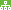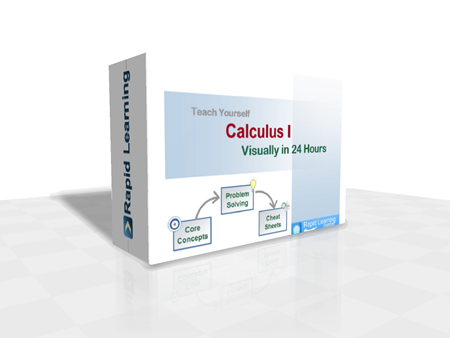How to Learn in 24 Hours?The Rapid Learning Movie

 Need Help? M-F: 9am-5pm(PST): Toll-Free: (877) RAPID-10 US Direct: (714) 692-2900 Int'l: 001-714-692-2900 24/7 Online Technical Support: The Rapid Support Center Secure Online Order:Need Proof? Testimonials by Our Users

 Rapid Learning Courses: Chemistry in 24 Hours Biology in 24 Hours Physics in 24 Hours Mathematics in 24 Hours Psychology in 24 Hours SAT in 24 Hours ACT in 24 Hours AP in 24 Hours CLEP in 24 Hours MCAT in 24 Hours (Medical) USMLE in 24 Hours (Boards) DAT in 24 Hours (Dental) OAT in 24 Hours (Optometry) PCAT in 24 Hours (Pharmacy) Nursing Entrance Exams Certification in 24 Hours eBook - Survival Kits Audiobooks (MP3)

 Tell-A-Friend: Have friends taking science and math courses too? Tell them about our rapid learning system.Home »  Mathematics » Calculus ICourse Preview

Calculus I Rapid Learning Series is the first of the three-course calculus sequence (I, II & III), designed typically for math, science and engineering majors or anyone who is required to take three semesters of calculus courses in college. This sequence of three courses cover the single variable and multivariable calculus. If you are required to take only one semester of calculus course, go for our College Calculus - RL411. If you are required to take three semesters of calculus courses, you should take our Calculus I, Calculus II and Calculus III, each represents one-semester of contents sequentially.

This Calculus I course includes elementary functions, trigonometry review, concepts of limits, derivatives, integrals and the applications, with the focus on conceptual understanding and problem solving.

With the understanding of the fact that for many students calculus is a difficult subject, we adapt a more gentle and visual approach to calculus concepts. This ensures the learning of calculus is easy and fun, by watching the YouTube like videos and playing interactive drills.

Starting with your basic understanding of functions and graphs, we introduce the concept of limits visually and build the foundation of calculus. For the core units of derivative and integral, we illustrate each concept graphically and demonstrate how to solve each type of calculus problems step by step. We then help to build your critical skills in problem solving, graphing calculators and calculus exam with visualization and examples.

1. 24 Core Concept Tutorials (Flash Movies)
24x visual tutorials for 24 chapters, with concept map, concept illustration, step-wise problem solving and topical summary, narrated by college calculus professors.
2. 24 Problem-Solving Drills (Flash Games)
24x feedback based Quizzes, concept-based problems, summary reviews on all problems and scoring system to track performance.
3. 24 Super Review Cheat Sheets (PDF Printables)
One cheat sheet per topic, key concepts in a single-sheet, at-a-glance review of each topic and both printable and laminatible Ideal for exam prep quick review.
Pre-requisites for Calculus: (1) Elementary Algebra - RL409 (2) Intermediate Algebra - RL407 (3) Pre-Calculus - RL403.

Calculus I Rapid Learning Series

This 24-chapter series provides comprehensive coverage of traditional college calculus topics for any students who are taking business calculus and calculus for life science, social science and other non-math majors.

Core Unit #1 – The Foundation

• Tutorial 01: Calculus I Overview
• Tutorial 02: Precalculus Review

Core Unit #2 – Functions and Graphs

• Tutorial 03: Functions and Analysis of Graphs
• Tutorial 04: Exponential Functions and Equations
• Tutorial 05: Inverse Functions and Logarithms

Core Unit #3 – Limits and Continuity

• Tutorial 06: The Concepts of Limits and Vertical Asymptotes
• Tutorial 07: Trigonometric Limits and Continuity
• Tutorial 08: Limits Involving Infinity
• Tutorial 09: Rates of Changes

Core Unit #4 – Derivatives

• Tutorial 10: The Tangent and Concept of Derivative
• Tutorial 11: Differentiability and Rules of Differentiation
• Tutorial 12: The Chain Rule and High Order Derivatives
• Tutorial 13: Implicit Differentiation and Related Rates
• Tutorial 14: Applications of Differentiation
• Tutorial 15: More Applications of Differentiation

Core Unit #5 – Integrals

• Tutorial 16: Riemann Sums and Definite Integrals
• Tutorial 17: The Fundamental Theorem of Calculus
• Tutorial 18: Antidifferentiation and Integration by Substitution
• Tutorial 19: Numerical Integration
• Tutorial 20: Applications of Integration
• Tutorial 21: Differential Equations and Mathematical Modeling

Core Unit #6 – Critical Skills

• Tutorial 22: Problem Solving in Calculus
• Tutorial 23: Graphing Utilities in Calculus
• Tutorial 24: Calculus Exam Guide

Each chapter comes with five learning components:
(1) Rich-Media Tutorials (Standard, Premium and Platinum Edition)
(2) Problem-Solving Drills (Standard, Premium and Platinum Edition)
(3) Super-Review Cheatsheets (Standard, Premium and Platinum Edition)
(4) Printable Tutorials (Premium and Platinum Edition)
(5) MP3 Audio Lectures (Platinum Edition Only)

Master Calculus the rich-media way, get started now:

Course Preview